BJ 5/28/09

edit 3/21/2017

Unit definitions

Brewing Vessel Calibration Constants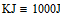A brewing vessel is calibrated by adding heated strike water to it and measuring the temperature decay of the water over time. This provides two calibration constants; the vessel's heat capacity, cv [J/K], and its heat loss coefficient, hlc [W/K].

The assumptions here are:

• The heat loss during the initial 5 minutes can be included into the vessel's heat capacity calculations
• The heat loss from 5 minutes on can be attributed to exponential heat loss through the vessel walls
• The specific heat of ingredients and the vessel's heat capacity are constant

Note that Mathcad uses implicit unit conversions, you may need to do this explicitly in your code.

Ambient

Water temperature at specific times (minutes)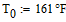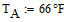Water volume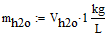Convert water volume to mass

Specific heat of water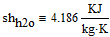Find Heat Capacity of Vessel

Initial Temperature of vesselStrike water temperatureEquilibrium temperature

Heat capacity of strike water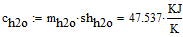Conservation of energy gives...Solving for vessel's heat capacitySanity check for equilibrium temperature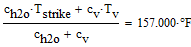Check

Characterize Temperature Decay

Time constant of temperature decay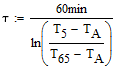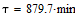Temperature vs. time function... in deg FPlot exponential temperature decay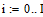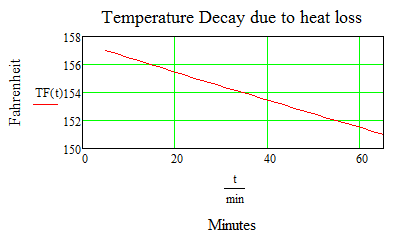(prevent solver from substituting values)

Find time derivative of temperature decay at the following time: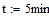Find Heat Loss Coefficient of Vessel

Power loss at T = T5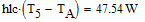Example on how to use the calibration constants

The calibration constants are used to select a strike water temperature to hit a target mash temperature. The example will also show what the temperature is at the end of the mash.

Mass of gristMash thicknessMash water volume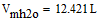Mass of mash water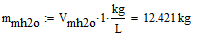Heat capacity of waterSpecific heat of gristHeat capacity of gristGrist temperature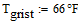Target mash temperature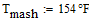Strike water temperature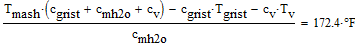Mash temp time constantMash timeTemperature at mash end